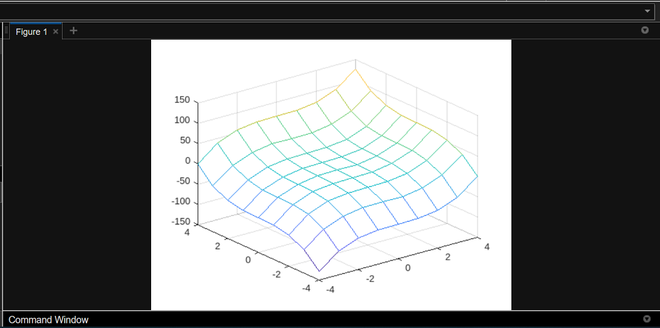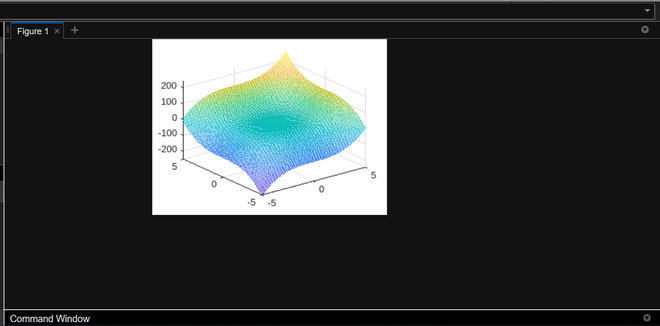Open in App
Not now

# How To Plot a Function of Two Variables in MATLAB?

• Last Updated : 14 Dec, 2022

In order to plot a function of two variables in Matlab first you have to know some functions like plot, meshgrid() function, and very well know about how to plot one variable functions in Matlab. You have to follow some main contents or you can say procedure to plot a function. Below is the process to plot two variables function in Matlab:

#### Algorithm:

• Define a grid of (x,y) points.
• Apply the function to the grid to get values of z.
• Use meshgrid() function.
• Plot the resulting mesh.

Let’s take an example to plot a two-variable function. Below is the plotting of the z= x^3 + y^3 function which is a two-variable function.

Example 1:

## Matlab

 `[x,y] = meshgrid(-4:1:4, -4:1:4);``z = x.^3 + y.^3;``mesh(x,y,z);`

Output:#### Explanation:

first, we make a grid that is -4 to 4 with a distance of 1 in both the x and y axis and then we calculated the z value by our equation x^3+y^3. and then we use the mesh function to plot x,y and z. If you want to see the surface of the function then below is a surface diagram of the above function:-

Example 2:

## Matlab

 `x = linspace(-5,5);``y = linspace(-5,5);``[x,y] = meshgrid(x,y);``z= x.^3 + y.^3;``mesh(x,y,z);`

Output:Explanation:

In the above example, we use linspace( x1,x2 ) function which will return a row vector of 100 evenly spaced points between x1 and x2 and then we calculated the z value which is x^3+y^3 at every point and then use mesh which plot in 3d surface.

My Personal Notes arrow_drop_up